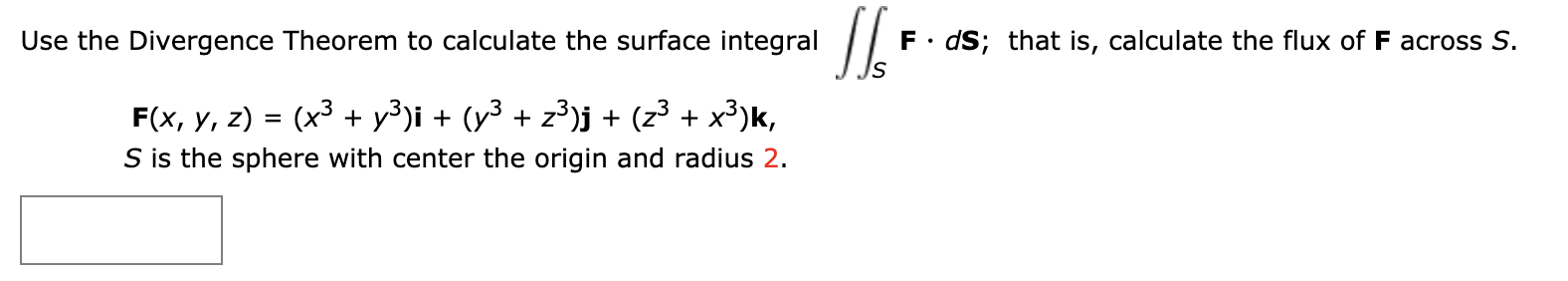# F dS; that is, calculate the flux of F across S. Use the Divergence Theorem to calculate the surface integral (x3 y3)i (y3 z3)j + (z3 + x3)k, F(x, у, 2) = S is the sphere with center the origin and radius 2.

Question

Use the Divergence Theorem to calculate the surface integral

 S
F · dS;

that is, calculate the flux of F across S.

F(x, y, z) = (x3 + y3)i + (y3 + z3)j + (z3 + x3)k,

S is the sphere with center the origin and radius 2.help_outlineImage TranscriptioncloseF dS; that is, calculate the flux of F across S. Use the Divergence Theorem to calculate the surface integral (x3 y3)i (y3 z3)j + (z3 + x3)k, F(x, у, 2) = S is the sphere with center the origin and radius 2. fullscreen